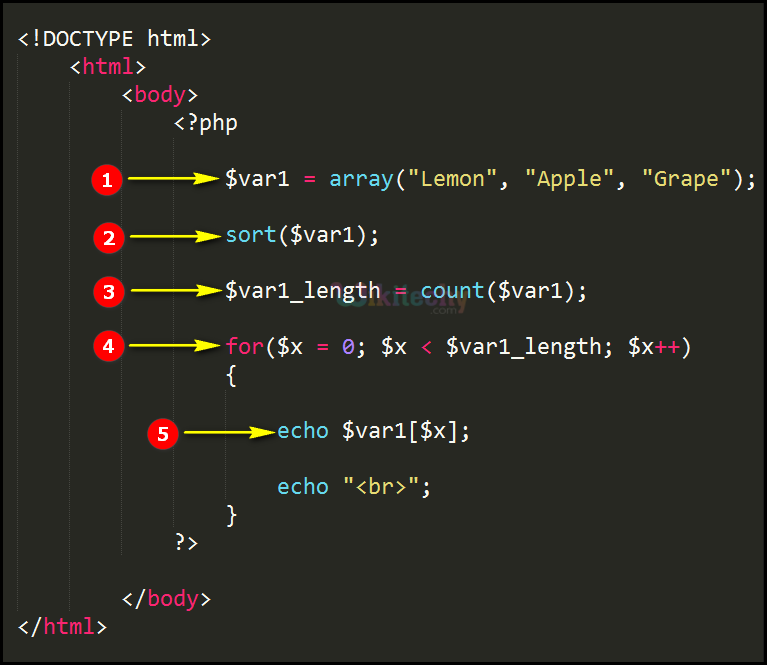# php tutorial - PHP Character Sort - php programming - learn php - php code - php script

• In php the sort function is used for sorting the array in terms of ascending order.
• Basically the characters are sorted in alphabetical order that is from A to Z Format.

## php examples Code Explanation :1. In this statement “\$var1” is an array variable having array values “Lemon, Apple and Grape”.
2. sort(\$var1); is the Sort function over here which is sorting the array values (Lemon, Apple and Grape) in ascending order.
3. In this statement the count function is used for counting the number of values in the array variable “var1” which is being stored in variable \$var1_length.
4. This statement will be defining the for loop statement for processing the array values.
5. echo \$var1[\$x]; This echo statement will print the sorted array values from the variable “\$var1” .

## php tutorials Sample Output :1. Here in this output the array values (Lemon, Apple and Grape) are shorted according to alphabetical order (Apple, Grape and Lemon) as shown here.